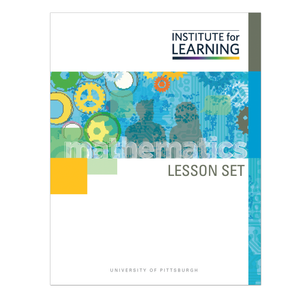# Proportional Relationships - Second Edition (Grade 7)

Regular price \$6.25 \$0.00 Unit price per

This lesson set provides a study of Proportional Relationships. Students will learn to move from the grade 6 study of ratios and equations of the form px = q to the study of proportional relationships. They combine their previous understanding of the topics of both ratio and equation to examine tests that establish or refute the presence of a proportional relationship between two variables. Students will:

• develop an understanding of proportional relationships;
• make and confirm conjectures about tests for proportionality;
• recognize proportional relationships visually when they are presented in equation or graphic form;
• recognize proportional relationships in tables and contexts; and
• articulate how to test for proportional relationships.

Standards: 7.RP.A.1, 7.RP.A.2, 7.RP.A.2.A, 7.RP.A.2.B, 7.RP.A.2.C